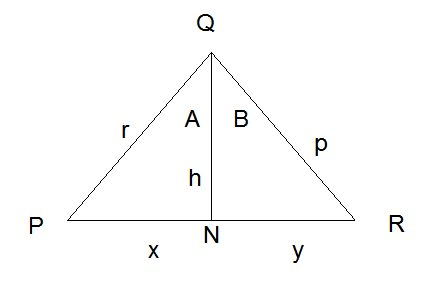# Proof “Area of Triangle” implies “Angle addition property for sin(x)”

This is a Challenge Question from my Intro to Set Theory Homework. I am to show

Given a triangle $\triangle$ ABC, with side lengths A,B,C and Corresponding angles $\alpha$,$\beta$,$c$. Prove that the following statement is true: $$Area(\triangle ABC)=\frac{1}{2}BC\sin(\alpha) \Rightarrow \sin(\alpha +\beta)=\sin(\alpha)\cos(\beta)+\sin(\beta)\cos(\alpha)$$

The Hint:

Compute the area of $\triangle$ABC is 2 different ways. You may use the usual area formula of a right triangle (it’s a special case of the formula you assume, anyway).

So I determined that "$\Rightarrow$" means that for all $\sin(\alpha +\beta)$, $\frac{1}{2}BC\sin(\alpha)$ must be true. So I can try to show that $\sin(\alpha +\beta)$ is a special case of $\frac{1}{2}BC\sin(\alpha)$.

And because of the hint. I was inclined to set the angle $\alpha$ to 90. Thus, $$\frac{1}{2}BC(1)\Rightarrow(1)\cos(\beta)+\sin(\beta)(0)$$ $$\frac{1}{2}BC\Rightarrow \cos(\beta)$$ $$\frac{1}{2}BC\Rightarrow\frac{C}{A}$$

And I'm left with a statement that doesn't mean anything, and frankly looks wrong. I am also unsure how to use the first part of the hint. The only other method I know for solving area of triangle is Heron's Law, which doesn't seem to fit. But, If I somehow got those two equations to equal, would it even prove the relation? or am I working in the wrong direction.

Any help or pointers are greatly appreciated.

• I don't understand why it has [set-theory] tag. – Hanul Jeon Jan 19 '17 at 8:41
• It's not set theory? The class that assigned this homework is called set theory – Jess L Jan 19 '17 at 19:22
• It is a question about Euclidean geometry. It does not related to any form of a set. – Hanul Jeon Jan 21 '17 at 7:19Proof:

$(1)$ Let $QN$ be a line that is perpendicular with $PR$.

$(2)$ Then $\text {area}_{\triangle PQR} = \text {area}_{\triangle PQN} +\text {area}_{\triangle RQN}$.

$(3)$ We have: $$\text {area}_{\triangle PQR} = (1/2)rp\sin(A+B)$$ $$\text {area}_{\triangle PQN} = (1/2)rh\sin(A)$$ $$\text {atea}_{\triangle RQN}= (1/2)ph\sin(B)$$

$(4)$ Combing $(2)$ and $(3)$ gives us: $$(1/2)rp\sin(A+B) = (1/2)rh\sin(A) + (1/2)ph\sin(B)$$ $$\Rightarrow rp\sin(A+B) = rh\sin(A) + ph\sin(B)$$ $$\Rightarrow \sin(A+B) = (\frac {h}{p})\sin(A) + (\frac {h}{r})\sin(B)$$

$(5)$ We also know $\cos B = \frac {h}{p}$ and $\cos A= \frac {h}{r}$.

$(6)$ So that we have: $$\sin(A+B) = \cos(B)\sin(A) +\cos(A)\sin(B)$$

Also $(a) \implies (b)$ means that if $(a)$ is true then $(b)$ must also be true. Hope it helps.